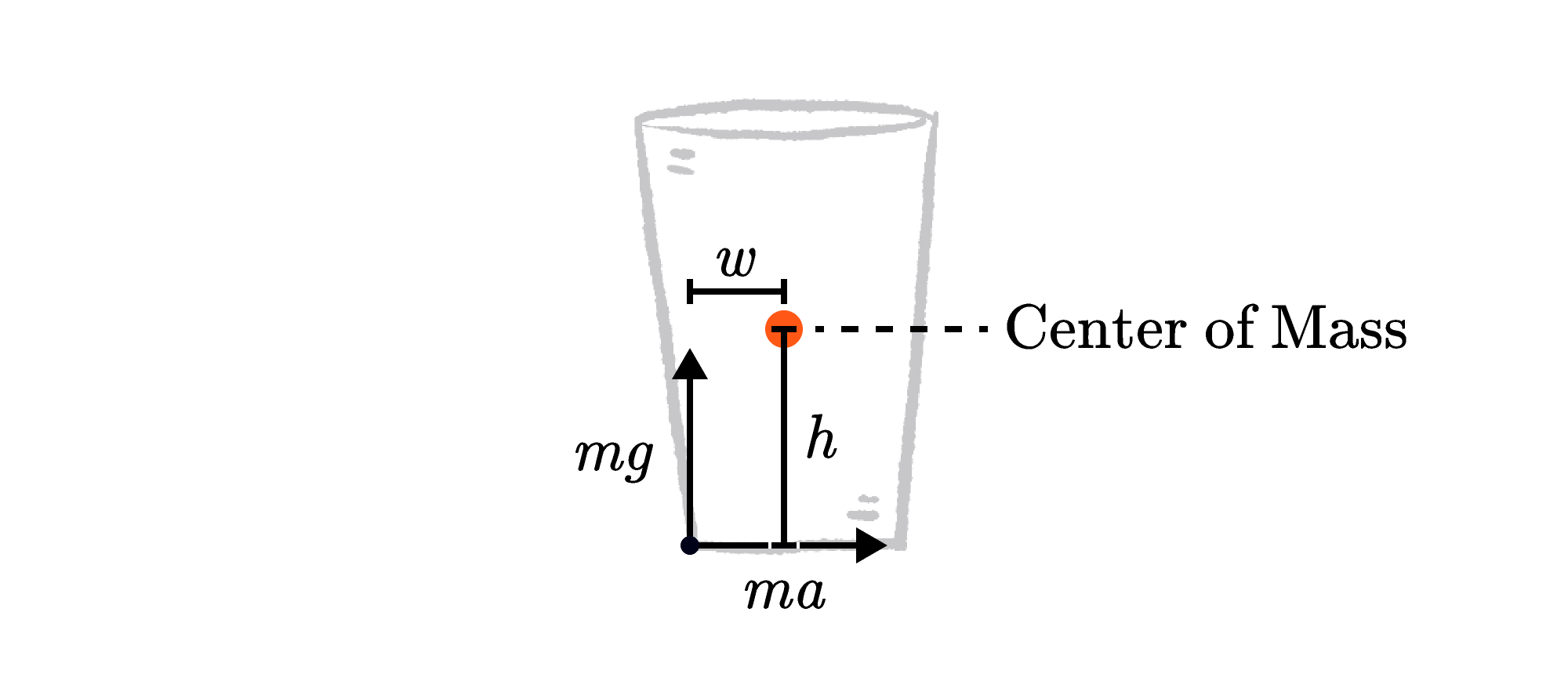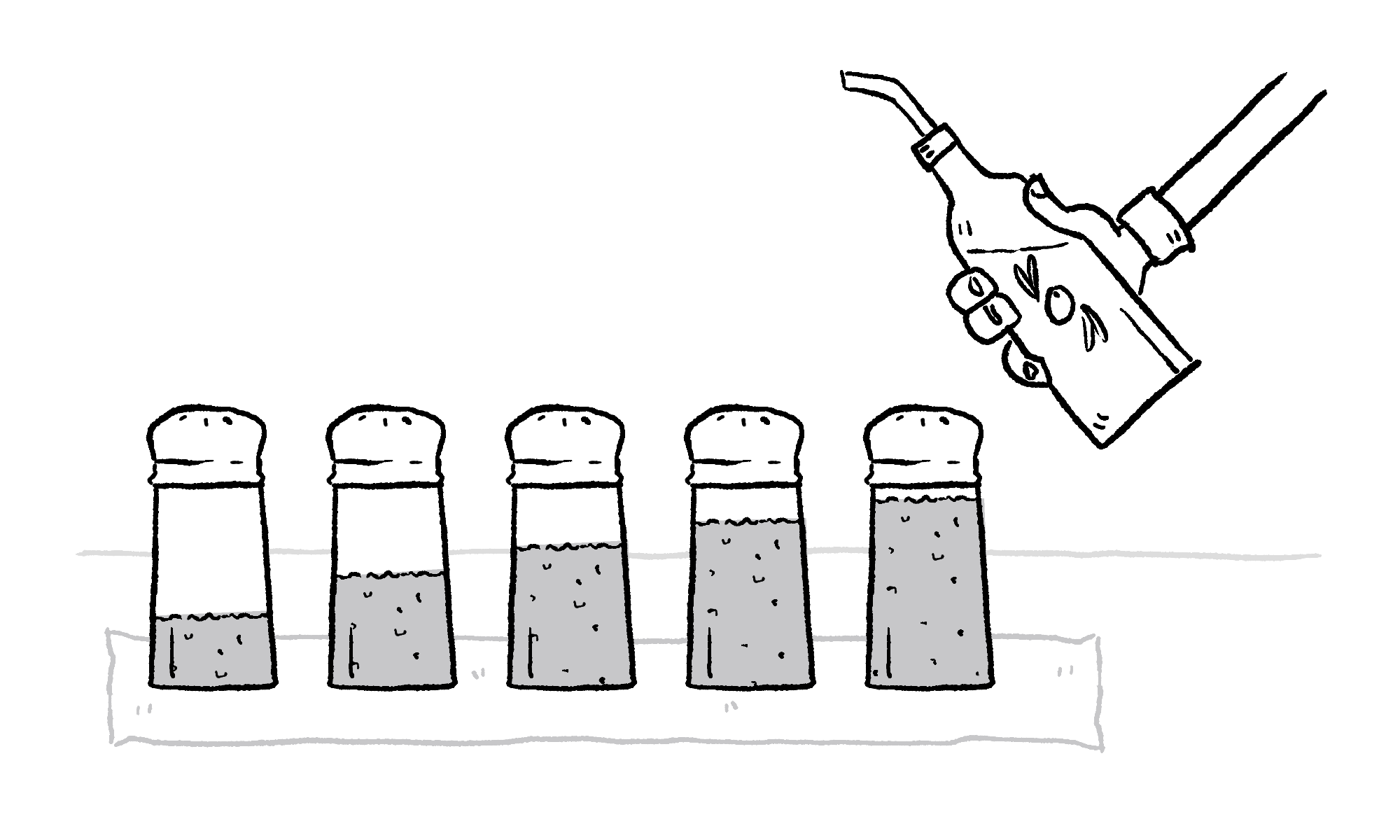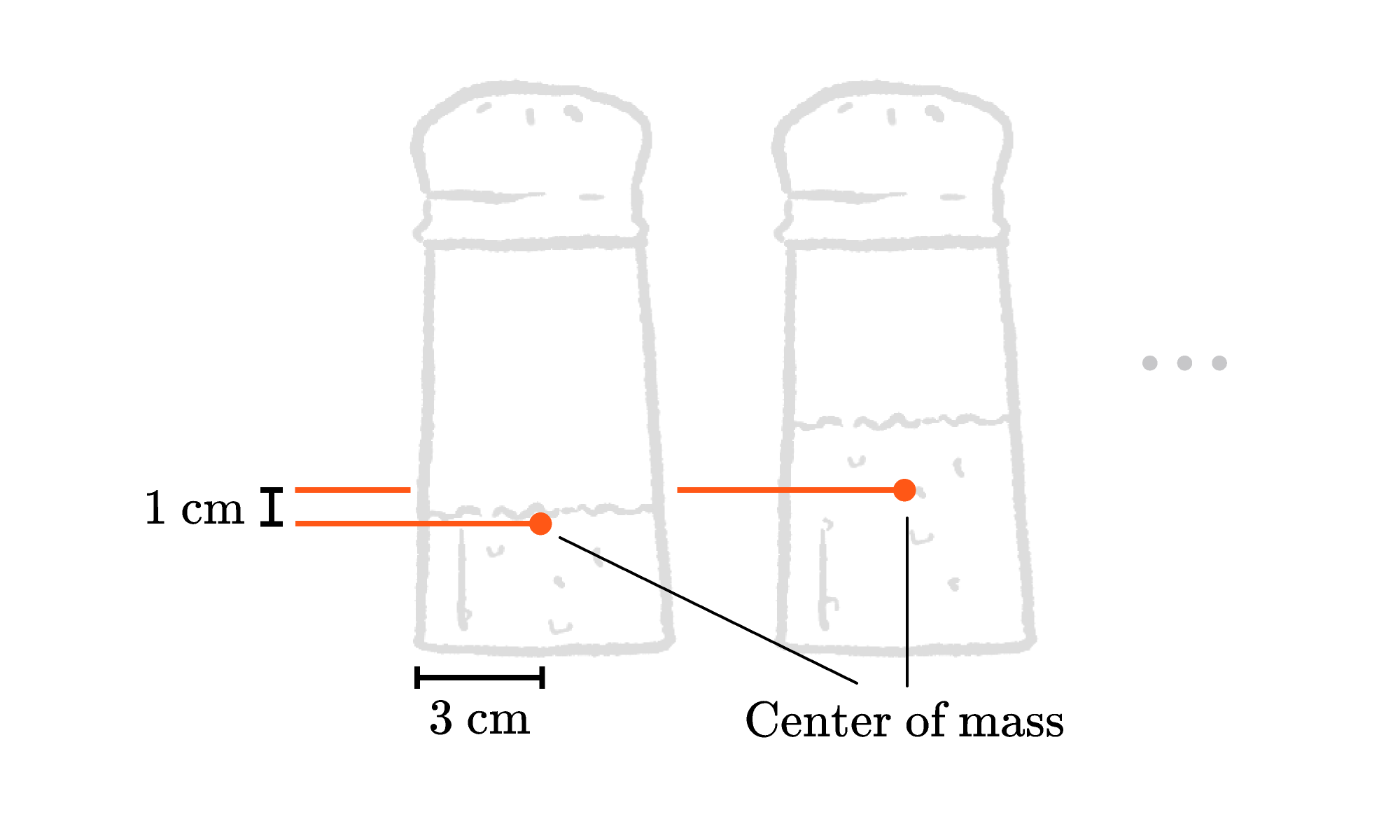Back

## Sugar Shenanigans

If you set a glass on a napkin and pull gently, the glass and the napkin should move together across the table. No big deal.

For a challenge, pull harder and try to slide the napkin out from under the glass — bonus points if you leave the glass standing!

Today's challenge can help you pinpoint the acceleration you need to remove the napkin successfully. Good luck! Post your attempts in the explanations — but any broken glassware is on you!

Anyone who has successfully pulled off the tablecloth trick knows the “sharpness” of your pull on the napkin or tablecloth is important. In other words, the answer depends on acceleration.

When the force you apply to the napkin is small, the acceleration you give it is also small and friction between the glass and the napkin can accelerate the glass at the same rate as the napkin.

If you give it a sharper tug, the dynamics play out differently. Either the napkin starts to slide out from under the glass, or the glass topples over leaving you with a mess. We'll deal with each of these outcomes in turn, starting with sliding.

When the glass accelerates with the napkin, Newton's law of motion says $F_\text{friction} = ma$, where $m$ is the mass of the glass and $a$ is the acceleration of the napkin. As long as the friction force is strong enough to match the acceleration you give the napkin, the napkin doesn't slide out. Sliding only begins when friction can't apply enough force to provide the necessary acceleration.

The maximum friction force $F_\text{friction, max}$ between the napkin and the glass depends on how firmly they're pressed together and a friction coefficient $\mu$ that tells you how grippy the materials are. Higher $\mu$ means friction is able to accelerate the glass more before it slides.

On a flat table, the firmness of their contact is gauged by the normal force $N,$ which on a flat table is just the weight of the glass, $N=mg.$ The constant $g$ is the gravitational acceleration $\SI[per-mode=symbol]{9.8}{\meter\per\second\squared}.$ Maximum friction is just the product of the normal force and the friction coefficient: $F_\text{friction, max}=mg\mu.$

Sliding happens when $ma \gt F_\text{friction, max}.$ Plugging in for $F_\text{friction, max},$ the mass cancels out and you find that the glass slides across the accelerating napkin when $a \gt g\mu.$ Once the glass starts to slide, friction will tend to drop a bit, making further sliding easier.

Toppling, you can probably intuit, is based in part on the glass’s geometry and how much liquid is in it — a tall, full glass with a narrow base is more likely to topple than a shorter, empty one.

On a flat surface, the weight of the glass acts on its center of mass, and the surface supports it with an equal and opposite normal force. As the napkin is pulled forward, the glass tends to rotate counterclockwise around the center of mass. If nothing negates this turning effect, the glass would immediately topple backwards.

However, the support force has the opposite turning effect — clockwise about the center of mass — and moves toward the back edge of the glass, much like the weight moving toward the back wheels of a vehicle when it accelerates suddenly.

But this support force can only move back so far — once the acceleration gets big enough that it reaches the back corner of the glass, it can go no further.

Therefore, the glass topples when the turning effect, or torque, of the accelerating force exceeds the torque of the support force when it’s acting at the back corner. Torque about the center of mass is calculated by the size of each force multiplied by its perpendicular distance from the center of mass, so the glass topples if $mah \gt mgw,$ where $h$ is the height of the center of mass and $w$ is the distance from the back edge of the glass.Canceling the mass of the glass, we find that toppling happens when $a \gt g \frac{w}{h}.$ Once again, it’s a simple number multiplied by the acceleration due to gravity.

But what if the acceleration of the paper is big enough for both sliding and toppling to happen? In this case, you should expect whichever happens at the lower acceleration to dominate. If the glass starts to slide first, then the accelerating force won’t get any bigger so the glass shouldn’t fall. If the glass starts to topple, it will keep falling even if it’s sliding as well!

So, what if you’re doing the trick with the tablecloth and want to leave something really tall behind? It isn’t impossible, but to make it slide before it topples you’ll need to minimize friction and make the center of mass as low as possible. So, get yourself a slippery tablecloth and a tall glass with a heavy base, rub some cooking oil on the bottom, and practice until you get the acceleration just right!

# Today's Challenge

You are practicing your tablecloth whisking with $5$ identical shakers, which have different amounts of sugar inside.

On your first attempt, you pull the cloth with just enough acceleration that all the shakers should slide. Unfortunately, four of them topple.Before your second attempt, you rub olive oil on the bottom of each shaker, reducing the friction coefficient from $\mu$ to $\frac{2}{3} \mu.$

Assuming you pull the cloth with the least possible acceleration that would cause all of the shakers to slide, how many shakers will definitely topple?

The center-of-mass heights of the shakers from left to right are $\SI{2}{\centi\meter},$ $\SI{3}{\centi\meter},$ $\SI{4}{\centi\meter},$ $\SI{5}{\centi\meter},$ and $\SI{6}{\centi\meter}.$ They have a radius of $\SI{3}{\centi\meter}.$×